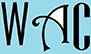## Geometry Question for Title 1 Schools: Length & Area Question?

9) If the length of a rectangle is twice the width of it, what is the length if the area is 72?

Area of a rectangle = length x width

Area for a rectangle “a” = length “l” x width “w”

Since “l” is twice “w”, then l = 2w

a =  l x w

72 = 2w x w

72 = 2w2

We have to make “w” subject of formula, so we move 2 to the other side.  When you move to the other side, addition becomes subtraction, multiplication becomes division, and vice versa.

72/2 = w(2 multiplied w at the other side)

36 = w2

6 = w

If the width is 6, then the length must be 12.